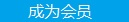您可以捐助，支持我们的公益事业。 1元 10元 50元 认证码：必填求知 文章 文库 Lib 视频 Code iProcess 课程 认证 咨询 工具 火云堂 讲座吧 成长之路要资料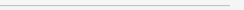订阅捐助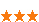2092 次浏览     评价： 好 中 差

Matplotlib是Python中最常用的可视化工具之一,可以非常方便地创建海量类型的2D图表和一些基本的3D图表。本文主要介绍了在学习Matplotlib时面临的一些挑战，为什么要使用Matplotlib，并推荐了一个学习使用Matplotlib的步骤。

matplotlib的新用户应该学习使用面向对象的接口。

matplotlib的另一个历史性挑战是，一些默认风格选项相当没有吸引力。 在R语言世界里，可以用ggplot生成一些相当酷的绘图，相比之下，matplotlib的选项看起来有点丑。令人欣慰的是matplotlib 2.0具有更美观的样式，以及非常便捷对可视化的内容进行主题化的能力。

1.学习基本的matplotlib术语，尤其是什么是图和坐标轴

2.始终使用面向对象的接口，从一开始就养成使用它的习惯

3.用基础的pandas绘图开始你的可视化学习

4.用seaborn进行更复杂的统计可视化

5.用matplotlib来定制pandas或者seaborn可视化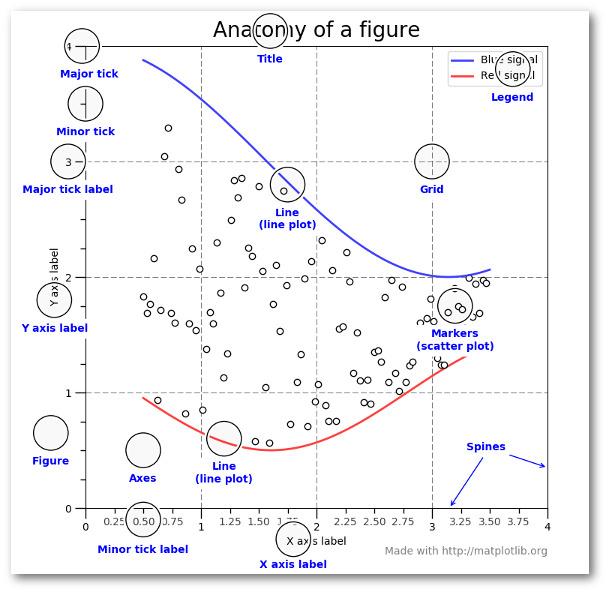import pandas as pd import matplotlib .pyplot as plt from matplotlib.ticker import FuncFormatter df = pd.read_excel("https://github.com/chris1610/ pbpython/blob/master/data/sample-salesv3.xlsx?raw=true") df.head()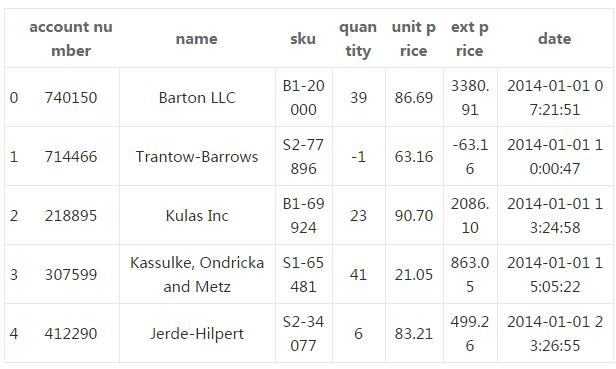top_10 = (df.groupby('name')['ext price', 'quantity'].agg({'ext price': 'sum', 'quantity' : 'count'}) .sort_values(by='ext price', ascending=False)) [:10].reset_index() top_10.rename(columns={'name': 'Name', 'ext price': 'Sales', 'quantity': 'Purchases'},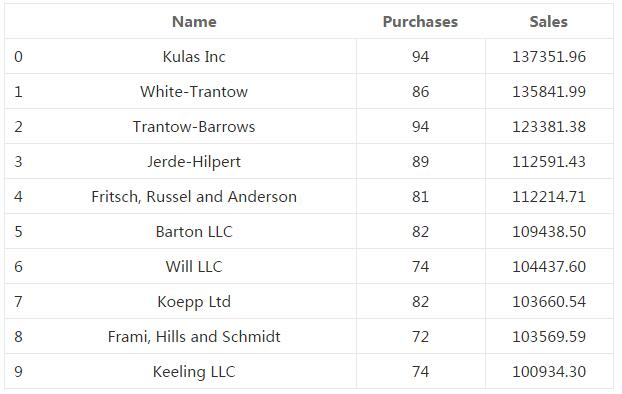plt.style.available
 ['seaborn-dark', 'seaborn-dark-palette', 'fivethirtyeight', 'seaborn-whitegrid', 'seaborn-darkgrid', 'seaborn', 'bmh', 'classic', 'seaborn-colorblind', 'seaborn-muted', 'seaborn-white', 'seaborn-talk', 'grayscale', 'dark_background', 'seaborn-deep', 'seaborn-bright', 'ggplot', 'seaborn-paper', 'seaborn-notebook', 'seaborn-poster', 'seaborn-ticks', 'seaborn-pastel']

 plt.style.use('ggplot')

 top_10.plot(kind='barh', y="Sales", x="Name")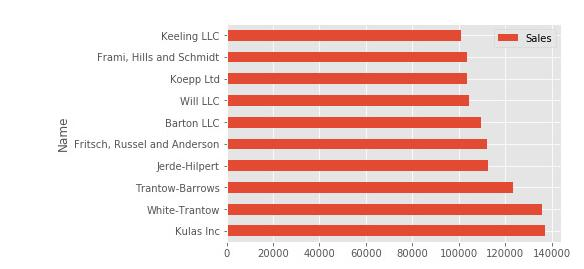fig, ax = plt.subplots() top_10.plot(kind='barh', y="Sales", x="Name", ax=ax)

 fig, ax = plt.subplots() top_10.plot(kind='barh', y="Sales", x="Name", ax=ax) ax.set_xlim([-10000, 140000]) ax.set_xlabel('Total Revenue') ax.set_ylabel('Customer');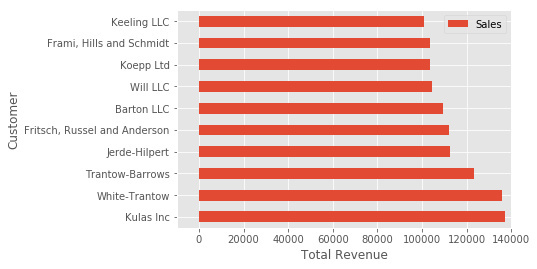Python fig, ax = plt.subplots() top_10.plot(kind='barh', y="Sales", x="Name", ax=ax) ax.set_xlim([-10000, 140000]) ax.set(title='2014 Revenue', xlabel='Total Revenue', ylabel='Customer') fig, ax = plt.subplots() top_10.plot(kind='barh', y="Sales", x="Name", ax=ax) ax.set_xlim([-10000, 140000]) ax.set(title='2014 Revenue', xlabel='Total Revenue', ylabel='Customer')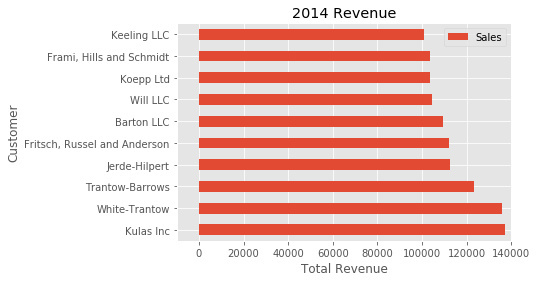fig, ax = plt.subplots(figsize=(5, 6)) top_10.plot(kind='barh', y="Sales", x="Name", ax=ax) ax.set_xlim([-10000, 140000]) ax.set(title='2014 Revenue', xlabel='Total Revenue') ax.legend().set_visible(False)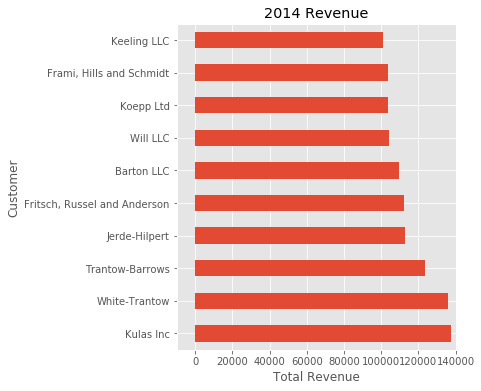def currency(x, pos): 'The two args are the value and tick position' if x >= 1000000: return '\${:1.1f}M'.format(x*1e-6) return '\${:1.0f}K'.format(x*1e-3)

 fig, ax = plt.subplots() top_10.plot(kind='barh', y="Sales", x="Name", ax=ax) ax.set_xlim([-10000, 140000]) ax.set(title='2014 Revenue', xlabel='Total Revenue', ylabel='Customer') formatter = FuncFormatter(currency) ax.xaxis.set_major_formatter(formatter) ax.legend().set_visible(False)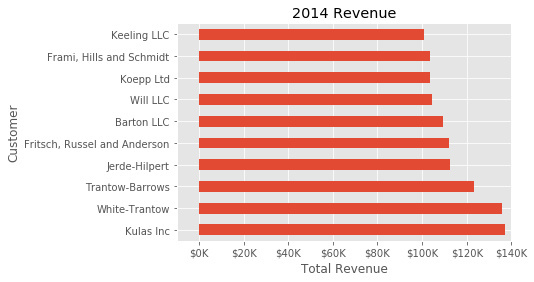Python # Create the figure and the axes fig, ax = plt.subplots() # Plot the data and get the averaged top_10.plot(kind='barh', y="Sales", x="Name", ax=ax) avg = top_10['Sales'].mean() # Set limits and labels ax.set_xlim([-10000, 140000]) ax.set(title='2014 Revenue', xlabel='Total Revenue', ylabel='Customer') # Add a line for the average ax.axvline(x=avg, color='b', label='Average', linestyle='--', linewidth=1) # Annotate the new customers for cust in [3, 5, 8]: ax.text(115000, cust, "New Customer") # Format the currency formatter = FuncFormatter(currency) ax.xaxis.set_major_formatter(formatter) # Hide the legend ax.legend().set_visible(False) # Create the figure and the axes fig, ax = plt.subplots() # Plot the data and get the averaged top_10.plot(kind='barh', y="Sales", x="Name", ax=ax) avg = top_10['Sales'].mean() # Set limits and labels ax.set_xlim([-10000, 140000]) ax.set(title='2014 Revenue', xlabel='Total Revenue', ylabel='Customer') # Add a line for the average ax.axvline(x=avg, color='b', label='Average', linestyle='--', linewidth=1) # Annotate the new customers for cust in [3, 5, 8]: ax.text(115000, cust, "New Customer") # Format the currency formatter = FuncFormatter(currency) ax.xaxis.set_major_formatter(formatter) # Hide the legend ax.legend().set_visible(False)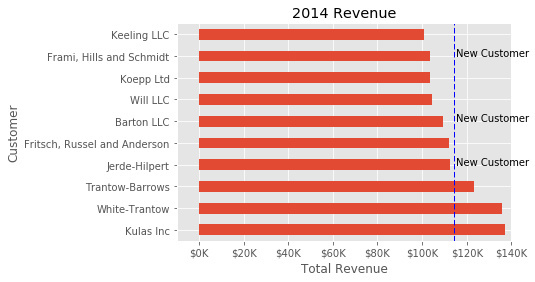fig, (ax0, ax1) = plt.subplots(nrows=1, ncols=2, sharey=True, figsize=(7, 4))

 # Get the figure and the axes fig, (ax0, ax1) = plt.subplots(nrows=1,ncols=2, sharey=True, figsize=(7, 4)) top_10.plot(kind='barh', y="Sales", x="Name", ax=ax0) ax0.set_xlim([-10000, 140000]) ax0.set(title='Revenue', xlabel='Total Revenue', ylabel='Customers') # Plot the average as a vertical line avg = top_10['Sales'].mean() ax0.axvline(x=avg, color='b', label='Average', linestyle='--', linewidth=1) # Repeat for the unit plot top_10.plot(kind='barh', y="Purchases", x="Name", ax=ax1) avg = top_10['Purchases'].mean() ax1.set(title='Units', xlabel='Total Units', ylabel='') ax1.axvline(x=avg, color='b', label='Average', linestyle='--', linewidth=1) # Title the figure fig.suptitle('2014 Sales Analysis', fontsize=14, fontweight='bold'); # Hide the legends ax1.legend().set_visible(False) ax0.legend().set_visible(False)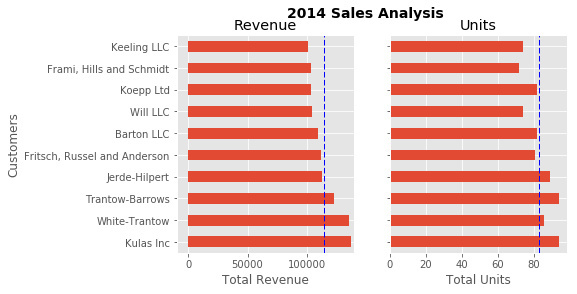Matplotlib支持许多不同格式文件的保存。 你可以用 fig . canvas . get _ supported _ filetypes（）查看系统支持的格式：

 fig.savefig('sales.png', transparent=False, dpi=80, bbox_inches="tight")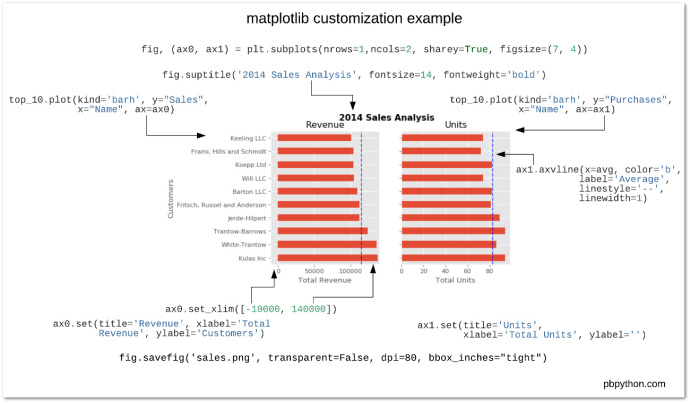2092 次浏览  评价: 好 中 差订阅捐助
 相关文章 手机软件测试用例设计实践 手机客户端UI测试分析 iPhone消息推送机制实现与探讨 Android手机开发（一）
 相关文档 Android_UI官方设计教程 手机开发平台介绍 android拍照及上传功能 Android讲义智能手机开发
 相关课程 Android高级移动应用程序 Android系统开发 Android应用开发 手机软件测试每天2个文档/视频 扫描微信二维码订阅
 订阅技术月刊 获得每月300个技术资源